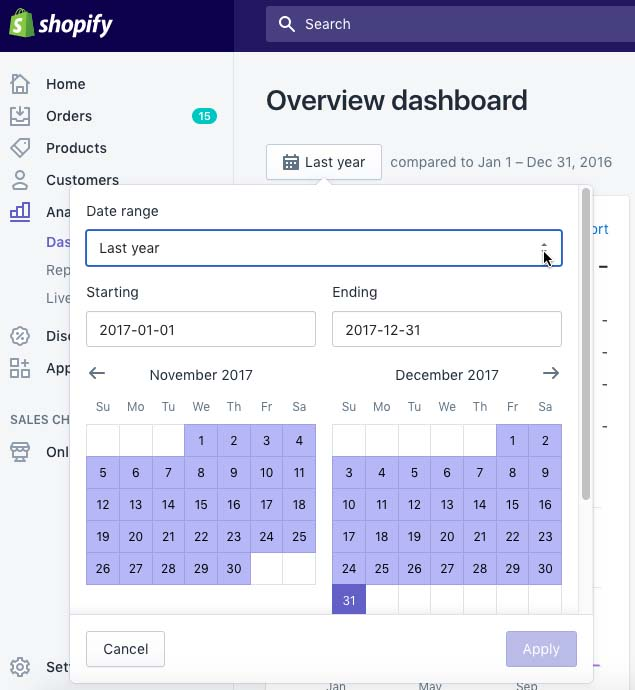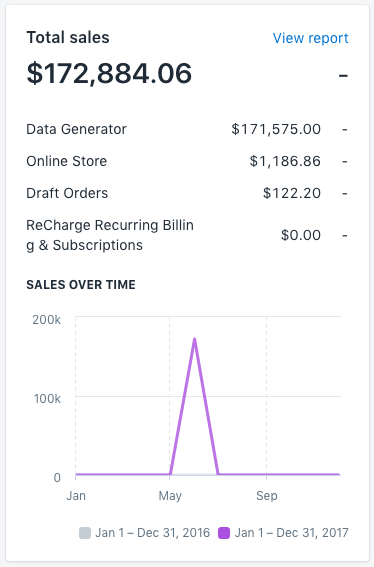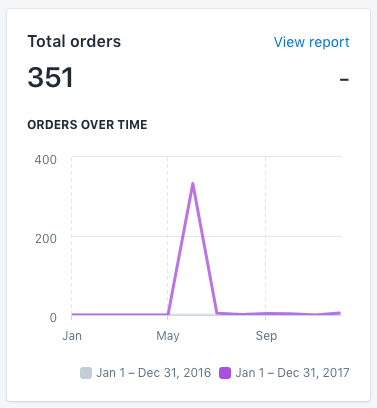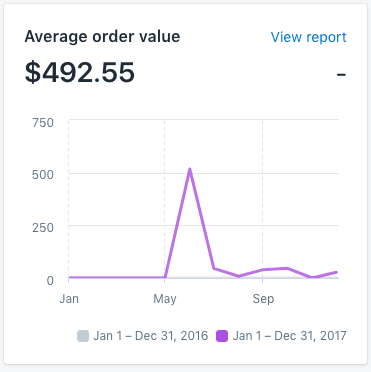# How to calculate average order value (AOV)

Last updated at:

## You will learn

Learn how to calculate your average order (AOV) or the total monetary value of your conversions (orders) divided by the number of conversions (orders that took place). Calculating your average order value (AOV) can be helpful when you want to add a condition to your segments to target high value or low value customers.

Note that AOV will only populate in Klaviyo if you have a Placed Order metric in your account.

## Calculating average order value

1. Use the calculation for AOV  as follows:

`Total store revenue / total number of orders`

2. If you want to calculate AOV for a certain time period, limit the timeframe for the numbers above to only capture revenue and orders within that period.

It's recommended to use the revenue and order values in your ecommerce site to calculate AOV. While Klaviyo does track this information, some customers that return products or cancel orders might cause slight discrepancies.

Here is an example of where to look for revenue and order information in a Shopify store.
2. Set the calendar dropdown to the time period you want to calculate for.3. On the Total Sales card find your total store revenue and save this locally.4. On the Total Orders card find your total orders amount and save this locally.5. Use the numbers from these two cards, along with the AOV equation above, to calculate your AOV. Use the value of your conversions (orders) divided by the number of conversions (orders that took place).

Also, note that some ecommerce platforms may include a report that specifically captures this number. You can use this to double check your own calculations.6. Once you have this number available, you can use this to create segments to analyze trends and send targeted messages based on your customers' shopping behavior.